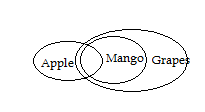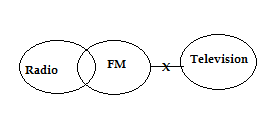# RRB ALP 2018 Practice Test Papers | Reasoning Questions (Day-79)

Dear Aspirants, Here we have given the Important RRB ALP & Technicians Exam 2018 Practice Test Papers. Candidates those who are preparing for RRB ALP 2018 can practice these Reasoning Questions to get more confidence to Crack RRB 2018 Examination.

[WpProQuiz 3115]

Click “Start Quiz” to attend these Questions and view Solutions

Direction: (1- 5) Read the following instruction and answer the question as per given set of series

2 4 62 3 4 7 6 5 8 9 0 7 6 5 7 5 7 9 5 3 5 6 8 9 1 2 4 6 1 5 6 4 6 3 1 3 4 5 6 2 1 3 5 6 2 6

1) How many odd numbers in the series are followed by square number and preceded by even number?

a) More than Three

b) One

c) Two

d) Three

2) Which digit of the series is fifteenth from the left of sixth from the right?

a) 1

b) 0

c) 2

d) 4

3) What is the resultant of the number 3rd digit from leftis divided by fifth from the right?

a) 2

b) 3

c) 4

d) 5

4) How many 2s are there followed by Cubic numbers (1 is also cubic number)?

a) One

b) Two

c) Three

d) None

5) If all odd number are dropped from this series, which of the following number is sixth from the right end?

a) 2

b) 4

c) 6

d) 8

Direction(6 – 10): The statement below is followed by two conclusions labeled I and II. Assuming That the Information in the statement is true, even if it appears at Variance with generally established facts, decide which conclusion(s) logically and definitely follow(s) from the information given in the statement.

6) Statement

Some Apple is mango

All Mangoes is Grape

Conclusion

I. Some mango is Grape

II. All Apples are Grape

a) Only I follow

b) Only II follow

c) None Follows

d) None of these

7) Statement

All FM is not Television

Conclusion

I. Some FM is Television is a possibility

II. Some Radio is television is a possibility

a) Both Follows

b) None follows

c) Only I follow

d) Only II follow

8) Statement

K=L ; J > L ; J < O

Conclusion

I. L < O

II. K < J

a) Both Follows

b) None follows

c) Only I follow

d) Only II follow

9) Statement

Y ≤ W; Y = P; Z ≥ Y

Conclusion

I. Z = P

II. Z > P

a) Both Follows

b) None follows

c) Only I follow

d) Either I or II Follows

10) Statement

Y ≤ W; Y = P; Z ≥ Y

Conclusion

I. W ≤ Y

II. W < Z

a) Both Follows

b) None follows

c) Only I follow

d) Either I or II Follows

Direction (1-5)

Fifth from the right = 3

Third from the left = 6

6/3 = 2

Direction (6-10)K = L < J < O

Z ≥ Y= P ≤ W